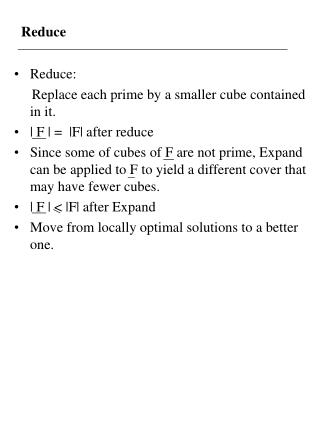DownloadDownload PresentationReduce

# Reduce

Download Presentation## Reduce

- - - - - - - - - - - - - - - - - - - - - - - - - - - E N D - - - - - - - - - - - - - - - - - - - - - - - - - - -
##### Presentation Transcript

1. Reduce • Reduce: Replace each prime by a smaller cube contained in it. • | F | = |F| after reduce • Since some of cubes of F are not prime, Expand can be applied to F to yield a different cover that may have fewer cubes. • | F | < |F| after Expand • Move from locally optimal solutions to a better one.

2. Reduce • Gives two possibilities for decreasing the size of cover - The reduced cube can be covered by a neighboring cube after the EXPAND. - The reduced cube can expand in different direction to cover some neighboring cube.

3. Reduce Definition: Smallest cube is a cube containing all minterms in not covered by D and = smallest cube containing ( ) = is still a cover.

4. Reduce Ci = SCC(Ci F(i)’ ) ?= SCC(Ci) SCC(F(i)’) Ex: F = C1 + C2 F(1) = F - C1= C2 SCC(F(1)’) = 1 ?= Ci SCC(F(i)’) c1 c2 C1 1 = C1 But, SCC(C1 F(1)’ ) = a single vertex x so C1 SCC(F(1)’ ) SCC(C1 F(1)’ )

5. Reduce Ci = SCC(Ci ) = Ci SCC( ) • SCC( ) • 1. Complementation • 2. Smallest cube containing problem • => Find a cube containing the complement • of a cover

6. Find a Cube Containing the Complement of a Cover • Proposition : The smallest cube c containing the complement of a cover F, F 1, satisfies I(C)i = 0 if xi 1 if xi’ 2 otherwise

7. Reduce F F xi = 0 xi = 1 xi = 0 xi = 1 (c)i = 0 (c)i = 2 F xi = 0 xi = 1 (c)i = 1 For general function, the above test employ difficult covering test. However, if it is a unate function, all test can be done easily.

8. Reduce Proposition : Let F be a unate cover. Then xi F if and only if there exists a cube, ci F, such that I(xi) I(ck) pf : A single output unate cover contains all primes of that function.

9. Reduce ex: x1 x2 x3 F 2 1 2 2 2 0 Find the smallest cube containing F’. x1 F x1’ F c1 = 2 x2 F c2 = 0 x3 ’ F c3 = 1 = ( 2 0 1)

10. Smallest Cube Containing Problem • Let • R-merge Let a and b be two cubes. Then, the smallest cube C containing {a, b} is a b , where denotes the coordinate wise union. ex: a = 0 1 2 1 b = 1 1 2 0 a b= 2 1 2 2

11. Reduce ex: F = 2 2 2 0 1 2 1 2 1 1 2 2 0 0 2 2 0 2 1 2 reduce C1 = 2 2 2 0 F(1) = 1 2 1 2 1 1 2 2 0 0 2 2 0 2 1 2 F(1)C1 = 1 2 1 2 1 1 2 2 0 0 2 2 0 2 1 2

12. Reduce {2 2 0 2} = SCC(F(1)C1’ ) 1 2 1 2 1 1 2 2 0 0 2 2 0 2 1 2 {0 1 0 2} {1 0 0 2} x1’ x1 2 0 2 2 2 2 1 2 2 2 1 2 2 1 2 2 x1’ {2 1 0 2} x1 {2 0 0 2} = SCC( ) c1 = 2 2 0 2 2 2 2 0 = 2 2 0 0

13. Reduce • order dependent • reduce the largest cube • reduce those cubes that are nearest to it. • compute pseudo distance (the number of mismatch) Ex: 0 1 0 1 0 2 1 1 PD = 2